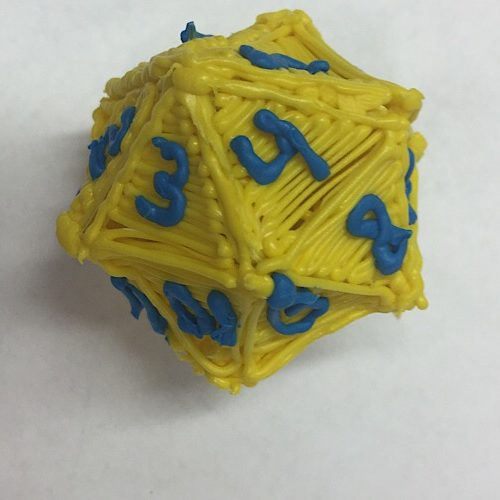### MATH: Doodler Dice Rollers

Time Required: Two 30-40- minute sessions
Skill Level: Intermediate

In this activity, students work in pairs to doodle dodecahedrons (12-sided) and icosahedrons (20-sided) to use in a math game that practices order of operations. Students will then create their own Doodle Dice Roller games to practice math skills.

Note: Any links outside of the3doodler.com are optional resources. We can’t ensure their upkeep or accuracy.### Lesson Plan

#### Instructions

Step 1

Students will work in pairs to doodle their own dodecahedron and icosahedron dice (one of each).

Step 2

Demonstrate how to use the stencils on the Doodle Rollers Dice Template in order to doodle each die. Model how to fold and then weld together each side of the polyhedra dice.

Step 3

Share the game: Doodler Dice Rollers. Explain the rules of the game. Students play in pairs or trios. Each player takes a turn rolling the three dice and creates an expression that includes three different operations, i.e., addition, multiplication, subtraction and division.

*Tailor this game to meet the ability level of your math students. The answer to each expression is recorded as the player's score for the round. The player with the highest score at the end of the game wins.

Step 4

Pass out pencils and lined paper for students to record each math expression, answers and scores for each round.

Step 5

Variation on the game: If a player watching notices a way to create a higher score with a different expression and the same numbers, that player gets to "steal" the score.

#### Wrap Up

Have the top class players face-off against one another. Instruct onlookers to try to beat each player's score by using the same numbers to create different math expressions each round.

#### Assessment

The teacher will assess students' understanding of order of operations by observing the mathematical expressions written by students as they play the game.

#### Possible Extensions

Students create their own Doodler Dice Games incorporating order of operations. Students will design a template for another type of polyhedra dice to use in new order of operations dice game.

#### Vocabulary

• algebra - a generalization of arithmetic in which letters representing numbers are combined according to the rules of arithmetic.

• collaboration - to work jointly with others or together especially in an intellectual endeavor.

• division - the mathematical operation of dividing something.

• geometry - a branch of mathematics that deals with the measurement, properties, and relationships of points, lines, angles, surfaces, and solids; broadly : the study of properties of given elements that remain invariant under specified transformations.

• math - the science of numbers and their operations, interrelations, combinations, generalizations, and abstractions and of space configurations and their structure, measurement, transformations, and generalizations.

• multiplication - a mathematical operation that at its simplest is an abbreviated process of adding an integer to zero a specified number of times and that is extended to other numbers in accordance with laws that are valid for integers.

• order of operations - The rules that say which calculation comes first in an expression.

• PEMDAS - an acronym for "Parenthesis, Exponents, Multiply, Divide, Add, Subtract," often remembered with the mnemonic device "Please Excuse My Dear Aunt Sally."

• prealgebra - a field of math in which the objective is to prepare students for the study of algebra.

• problem-solving - the process or act of finding a solution to a problem.

• subtraction - the operation of deducting one number from another.

#### Educational Standards

Common Core
CCSS.MATH.CONTENT.5.OA.A.1

Use parentheses, brackets, or braces in numerical expressions, and evaluate expressions with these symbols.

In This Lesson

Students will compose and evaluate their own mathematical expressions incorporating three different types of operations and implementing parentheses, brackets or braces where needed during the Doodler Dice Rollers Game.

Common Core
CCSS.MATH.CONTENT.5.OA.A.2

Write simple expressions that record calculations with numbers.

In This Lesson

Students will compose simple expressions that record calculations with numbers during the Doodler Dice Rollers Game.

CS Teachers
1A-A- 3-5

Decompose (break down) a larger problem into smaller sub-problems with teacher guidance or independently.

In This Lesson

Students will break down the process of understanding the order of operations by designing their own polyhedra dice, playing a game the includes multiple operations and then designing their own dice games based on order of operations.

ISTE
1C

Use technology to seek feedback that informs and improves their practice and to demonstrate their learning in a variety of ways.

In This Lesson

Students will use the 3Doodler to doodle polyhedra dice.

ISTE
6B

Create original works or responsibly repurpose or remix digital resources into new creations.

In This Lesson

Students will use a 3Doodler to doodle polyhedra dice.

ISTE
7A

Use collaborative technologies to work with others, including peers, experts or community members, to examine issues and problems from multiple viewpoints.

In This Lesson

Students will seek feedback from a partner during all stages of the process for this activity and the extension activity.

Back to Lessons